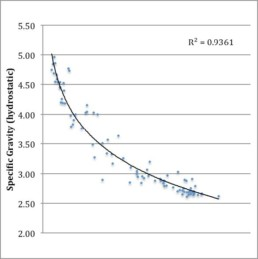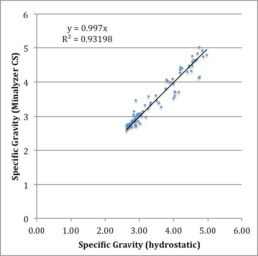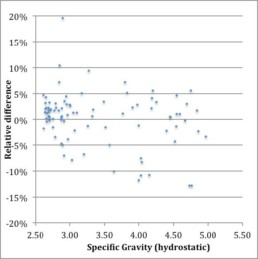# Specific Gravity Estimation with Minalyzer CS

### Case Study

In exploration and mining it is important to know the density or specific gravity of rocks. Chemical analyses only report concentrations as a percentage of weight. In order to calculate the tonnage of an  ore  body,  the  commodity  weight  percent  has  to  be  multiplied  by  the  rock  density.  After  all,  copper and iron are sold per ton!

A  common  but  rather  labour  intensive  method  is  hydrostatic  weighing.  The  specific  gravity  is  calculated according to Archimedes’s Principle by weighing the rock separately in air and water. The difference  in  measured  weight  is  proportional  to  the  buoyancy  of  the  displaced  water.  Thus  the  specific gravity can be calculated relative to water (density ≈ 1 g/cm3).

Sensors in Minalyzer CS allow accurate estimation of the specific gravity of rocks, simultaneous with chemical analysis. This means that along with the XRF analytical results, there is an estimate of the specific  gravity  for  each  analysis.  Calibration  of  the  Specific  Gravity  curve  is  done  by  measuring  samples with known specific gravity.After  applying  the  calibration  curve,  the  relation  between  the  hydrostatic  specific  gravity  and  Minalyzer CS specific gravity is a linear 1 : 1 ratio.Relative  differences  between  the  hydrostatic  specific  gravity  and  the  estimated  specific  gravity  by  Minalyzer CS are generally less than ±10 %.